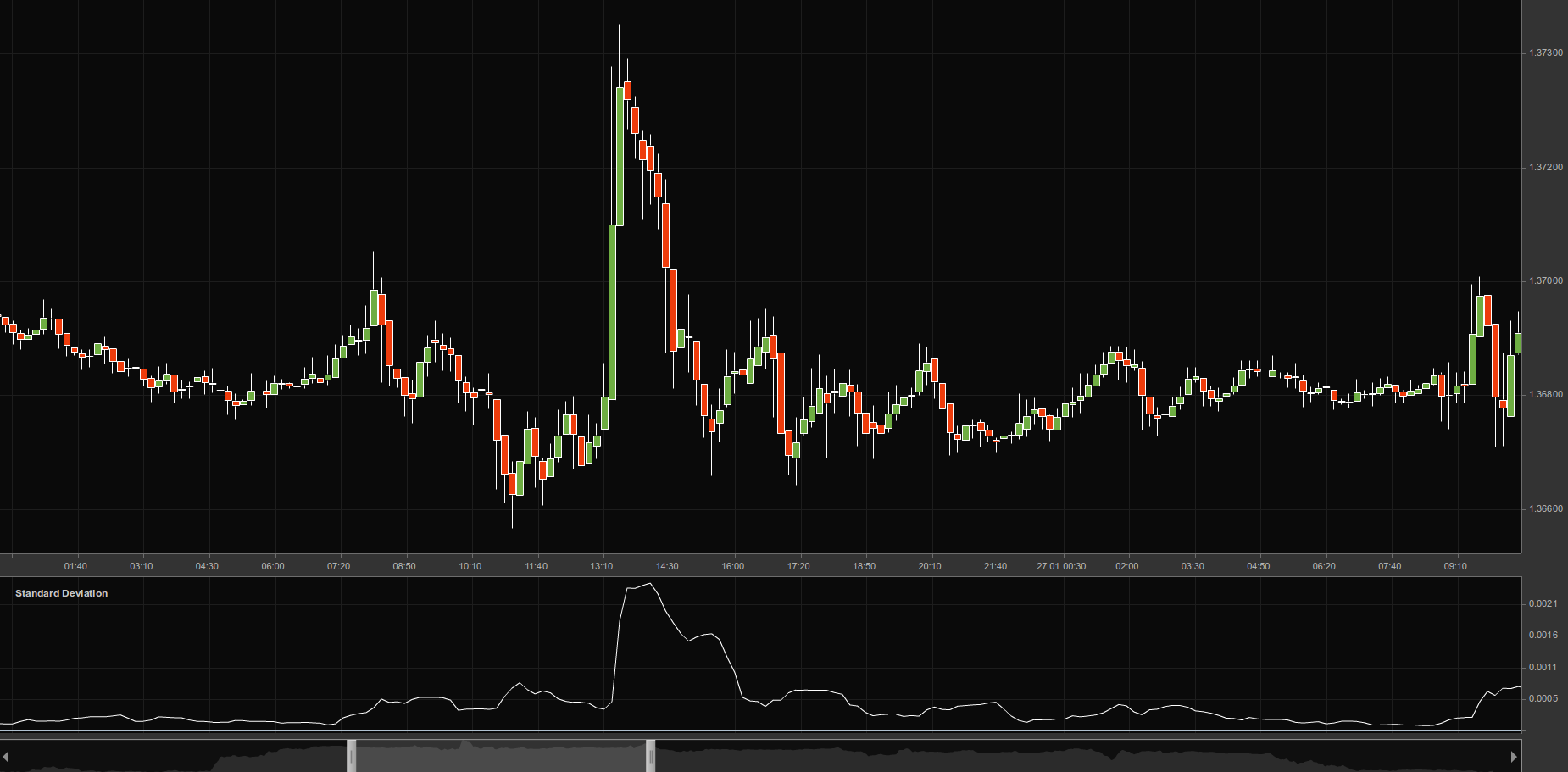# Standard Deviation Indicator

## Standard Deviation Indicator

### This lesson will cover the following

• What is Standard Deviation Indicator
• How is it visualized
• What is its use
• When should it be used

Standard deviation is an indicator that measures the size of an assets recent price moves in order to predict how volatile the price may be in the future.

It can help you decide whether volatility is likely to increase or decrease. A very high standard deviation reading indicates that a huge price change has just occurred, but that a decrease in volatility could soon follow. A very low standard deviation reading indicates the opposite.

## Best Forex Brokers for United StatesThe standard deviation indicator is part of the calculation of Bollinger bands, and is also practically synonymous with volatility.

This indicator measures the scale of price deviation related to the moving average. This means that if the indicators value is large, the market is experiencing high volatility and candlesticks are rather dispersed around. Conversely, if the value is smaller, then market volatility is low and prices are rather close to the moving average.

The standard deviation indicator is easy to interpret since it reflects market behavior, which itself consists of shifts between highly active and sluggish market conditions. If standard deviation is too low, i.e. the market is exceedingly inactive, it would make sense to expect a spike soon. Conversely, if the value is extremely high, then a decline in activity is likely about to follow.## Use of the Standard Deviation Indicator

Using the probability distribution models allows you to create many trading strategies, but the most common use of the standard deviation indicator is to predict price reversals based on the principle of reversion to the mean.

Retracement to the average is basically the principle on which oscillators like the Relative Strength Index are constructed. It argues that each deviation from the mean must be followed by a return to the same so that the overall distribution of prices fits the standard distribution.

## When to use standard deviation

Standard deviation is considered as one of the most reliable indicators available to traders, but under certain conditions. In trending markets where volatility is moderate and price oscillation is concentrated around the middle of the range, the standard deviation indicator is one of the best tools you would find. Many of the methods hedge fund operators and bank analysts use are strongly dependent on normal distribution patterns.For example, if a currency is oscillating between 1.2700 and 1.3700 for an extended period of time, with much of the movement bound in the middle of the range, you can trade the pattern by assuming mean regression on the basis of standard distribution.

Conversely, if prices are clustered at the edges of the same range, for example, around 1.3600-1.3700, or 1.2700 and 1.2800, the probability distribution of the prices may not be standard and using the standard deviation indicator for trading while assuming mean regression may be disastrous.

And this is quite important because it is one of the main drawbacks when trading moving averages in general as well. The average of prices will be the same in both a pattern where prices are concentrated predominantly at the edges of the range and one where they are focused in the middle. However, these two patterns obey completely different rules. Therefore, you shouldnt apply the same mean regression strategy based on a single basic reading of market movement. You need to first analyze the distribution of prices, the range and the long-term trend in which they exist in order to apply the standard deviation indicator correctly.